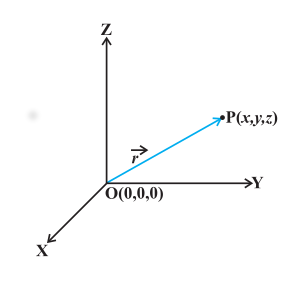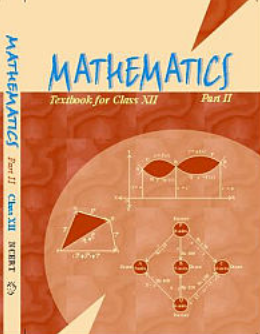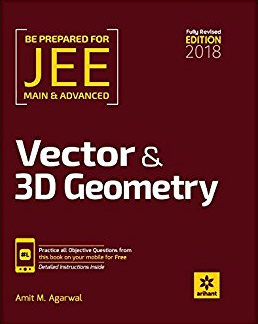# Vector Algebra   Share

## What is Vector Algebra

Vector Algebra is defined as the mathematical operations done on vectors, which is further the foundation of modern-day 3D gaming, animation and widely used in modern physics. At the JEE level, Vector Algebra is simple to understand. Every year you will get 1 - 2 questions in JEE Main exam as well as in other engineering entrance exams. This chapter helps you in 3-D Geometry and Physics (Kinematics, Work, Energy and Power, electrostatics, etc.). Importance of this chapter can be seen from Physics where you learn why any quantity is scalar or vector? A little mistake in vector algebra costs you negative marks. As compared to other chapters in maths, Vector Algebra requires high accuracy to prepare for the examination. Once you start learning vector algebra you will become familiar with the application of vectors and it helps you to solve the problems based on 3-D geometry and basics used in physics.

Suppose you are playing hide n seek game and one of your friends gives you a hint that he is 5 steps away from you. Would you be able to catch him??

This will take you many more tries as you are not aware of the direction.

Suppose, he is behind you and you start moving in the forward direction. What happened?? Distance will start increasing between both of you.

What if he tells you that he is in front of you?

Now you can easily catch him as you know the direction as well as the magnitude of the distance of your friend. That's how we make use of vectors in real life unknowingly.

There are many more examples like computing the direction of rain, flight of a bird and the list is never-ending.

Prepare Online for JEE Main/NEET

Crack JEE 2021 with JEE/NEET Online Preparation Program

Well, all the answers to these questions you will able to tell when you study Vector Algebra.

After reading this chapter  you will be able to:

• Determine the position of any object

• Represent the position of the object w.r.t the another object

• Determine the least possible way to reach one position w.r.t the other position

## Notes  of Vector Algebra

Important Topics of Vector Algebra

• Vector (Position Vector, Direction cosine)

• Types of vector

• Vector Algebra (Addition of vectors and multiplication of vector with scalar)

• Section Formula

• Product of two vectors (Scalar product and Vector product)

## Overview of Chapter

Vector- In general terms, a vector is defined as an object having both directions as well as magnitude.

Position vector- Consider a point P in space, having coordinates (x, y, z) with respect to the origin O (0, 0, 0). Then, the vector  having O and P as its initial and terminal points, respectively, is called the position vector of the point P with respect to O.Direction Cosines- The position vector of a point P(x, y, z). The angle α, β, and γ made by the vector with the positive direction of x, y, and z-axes respectively, are called its directions angles. The cosine values of these angles, i.e., cos α, cos β, and cos γ are called direction cosines of the vector and usually denoted by l, m, and n, respectively.## Types of vector

Zero Vector: A vector whose initial and terminal points coincide, is called a zero vector (or null vector). Zero vector can not be assigned a definite direction as it has zero magnitudes. Or, alternatively, otherwise, it may be regarded as having any direction. The vector  represents the zero vector.

Unit Vector: A vector whose magnitude is unity (i.e., 1 unit) is called a unit vector. The unit vector in the direction of a given vector  is denoted by â.

Coinitial Vectors: Two or more vectors having the same initial point are called coinitial Vectors.

Collinear Vectors: Two or more vectors are said to be collinear if they are parallel to the same line, irrespective of their magnitudes and directions.

Equal Vectors: Two vectors are said to be equal, if they have the same magnitude and direction regardless of the positions of their initial points, and written as .

Negative of a Vector: A vector whose magnitude is the same as that of a given vector (say,), but the direction is opposite to that of it, is called negative of the given vector.

Addition of two vectors simply means displacement from a point A to point B.

In general, if we have two vectors . Then  resultant  of two vector is,

Points to be remember

• Triangle law of vector addition

• Parallelogram law of vector addition

## Multiplication of vector with a scalar

The product of the vector  by the scalar λ, is called the multiplication of a vector by the scalar λ and denoted as λ

And vector λ is collinear to the vector

The vector λ has the direction same or opposite to that of vector  according to the value of λ (same direction for positive value and vice versa). Also, the magnitude of vector λ is | λ | times the magnitude of the vector , i.e., | λ | = | λ | ||.

## Component Form of Vector

If a vector is represented as   then it is called component form. Here, representing the unit vectors along the

x, y, and z-axes, respectively and (x,y,z)  represent coordinates of the vector.

Some important points

If  are any two vectors given in the component form  and  , respectively, then,

• The resultant of the vectors is

• The vectors are equal if and only if

• The multiplication of vector \vec{a} by any scalar λ is given by

## Section Formula - Vector Algebra

When point R divides \vec{PQ} internally in the ratio of m:n such that then .

When point R divides externally in the ratio of m:n such that then .

## Product of two vector

Multiplication of two vectors is defined in two ways (i) Scalar (or dot) product and (ii) Vector (or cross) product.

In scalar product resultant is scalar quantity.

In vector product resultant is a vector quantity.

Scalar product

Scalar product of two non zero vectors is denoted by . Scalar product is calculated as where, θ is the angle between two non zero given vectors.

Projection of a vector

Vector product

Vector product of two non zero vectors is denoted by. Scalar product is calculated as where θ is the angle between two non zero vectors and \hat{n} is a unit vector perpendicular to both.

## How to prepare Vector Algebra?

Vector Algebra is one of the basic topics, you can prepare this topic by understanding a few basic concepts

• Start with the basic concept of vector, understand all the terms used in vector algebra.

• Representation of a vector is an important part of this chapter. It is important for you that you should read all the questions meditatively.

• Vector is all about the direction with magnitude so make sure that the direction given in question and direction obtained in answer match properly.

• Make sure that after studying certain section/concept, solve questions related to those concepts without looking into the solutions and practice MCQ from the above-mentioned books and solve all the previous year problems asked in JEE.

• Don’t let any doubt remain in your mind and clear all the doubts with your teachers or with your friends.

## Best Books For Preparation:-

First, finish all the concepts, example and questions given in NCERT Maths Book. You must thorough with the theory of NCERT. Then you can refer to the book Cengage Mathematics Algebra. Vector Algebra is explained very well in this book and there are ample amount of questions with crystal clear concepts. You can also refer to the book Arihant Algebra by SK Goyal or RD Sharma. But again the choice of reference book depends on person to person, find the book that best suits you the best depending on how well you are clear with the concepts and the difficulty of the questions you require.

## Maths Chapter-wise Notes for Engineering exams

 Chapters Chapters Name Chapter 1 Sets, Relations, and Functions Chapter 2 Complex Numbers and Quadratic Equations Chapter 3 Matrices and Determinants Chapter 4 Permutations and Combinations Chapter 5 Binomial Theorem and its Simple Applications Chapter 6 Sequence and Series Chapter 7 Limit, Continuity, and Differentiability Chapter 8 Integral Calculus Chapter 9 Differential Equations Chapter 10 Coordinate Geometry Chapter 11 Three Dimensional Geometry Chapter 13 Statistics and Probability Chapter 14 Trigonometry Chapter 15 Mathematical Reasoning Chapter 16 Mathematical Induction

### Topics from Vector Algebra

• Vectors and scalars, addition of vectors, components of a vector in two dimensions and three dimensional space ( AEEE, JEE Main, SRMJEEE, TS EAMCET, VITEEE, AP EAMCET, COMEDK UGET ) (238 concepts)
• Scalar and vector products, scalar and vector triple product ( AEEE, JEE Main, SRMJEEE, TS EAMCET, VITEEE, AP EAMCET, COMEDK UGET ) (490 concepts)
• Introduction to 3-D Geometry ( AEEE, JEE Main, SRMJEEE, TS EAMCET, VITEEE, AP EAMCET, COMEDK UGET ) (6 concepts)
• Vector Algebra ( AEEE, JEE Main, SRMJEEE, TS EAMCET, VITEEE, AP EAMCET, COMEDK UGET ) (54 concepts)
• Scalar and Vector Product of Vector Algebra ( AEEE, JEE Main, SRMJEEE, TS EAMCET, VITEEE, AP EAMCET, COMEDK UGET ) (66 concepts)

### Important Books for Vector Algebra

••Exams
Articles
Questions